# How to Solve for the Moment of Inertia of Irregular or Compound Shapes

• Author:
• Updated date:

Ray is a licensed engineer in the Philippines. He loves to write about mathematics and civil engineering.

## What Is Moment of Inertia?

Moment of Inertia also called as "Angular Mass or Rotational Inertia" and "Second Moment of Area" is the inertia of a rotating body with respect to its rotation. Moment of Inertia applied to areas has no real meaning when examined by itself. It is merely a mathematical expression usually denoted by symbol I. However, when used in applications like flexural stresses in beams, it begins to have significance. The mathematical definition moment of inertia indicates that an area is divided into small parts dA, and each area is multiplied by the square of its moment arm about the reference axis.

I = ∫ ρ2 dA

The notation ρ (rho) corresponds to the coordinates of the center of differential area dA.

## Step-By-Step Procedure in Solving for the Moment of Inertia of Composite or Irregular Shapes

1. Identify the x-axis and y-axis of the complex figure. If not given, create your axes by drawing the x-axis and y-axis on the boundaries of the figure.

2. Identify and divide the complex shape into basic shapes for easier computation of moment of inertia. When solving for the moment of inertia of a composite area, divide the composite area into basic geometric elements (rectangle, circle, triangle, etc) for which the moments of inertia are known. You can show the division by drawing solid or broken lines across the irregular shape. Label each basic shape to prevent confusion and miscalculations. An example is shown below.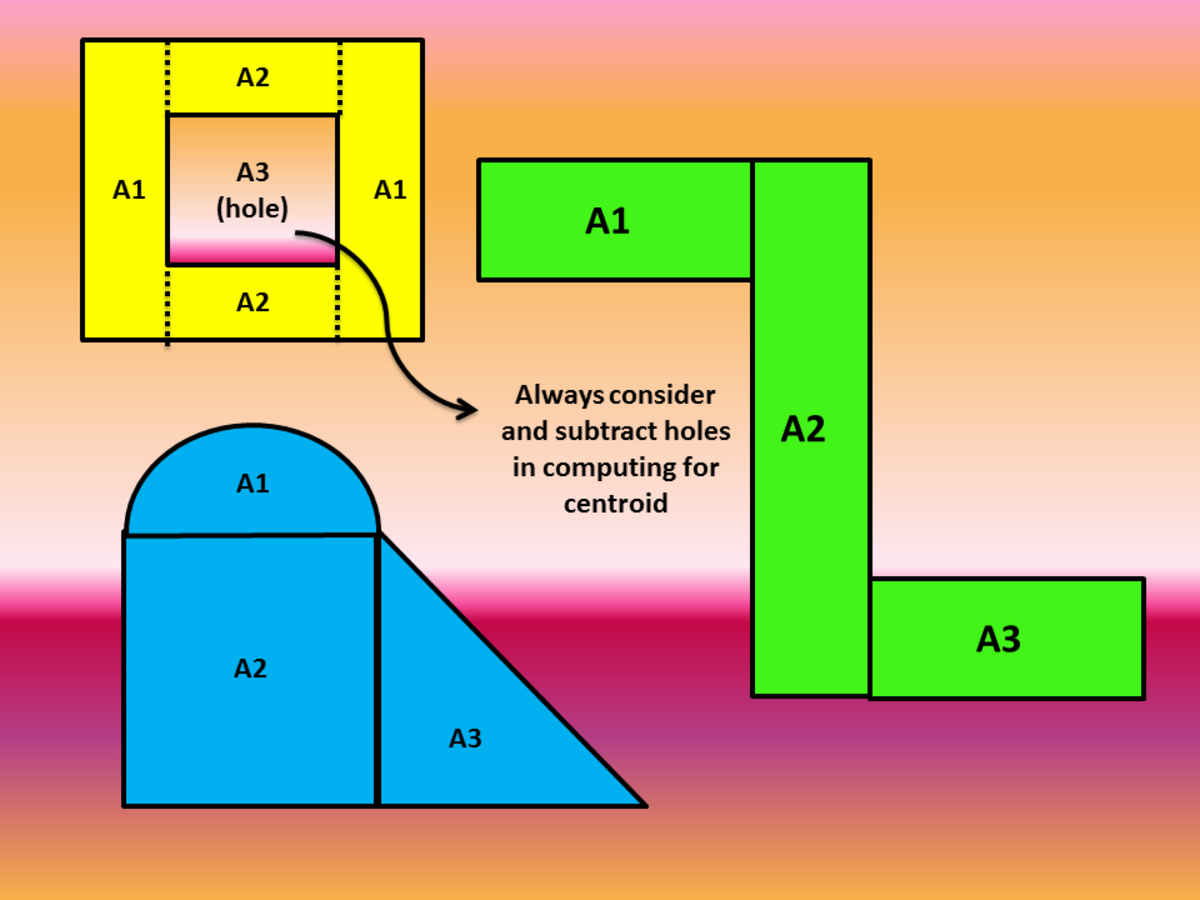Division of Basic Shapes in Solving for Moment of InertiaJohn Ray Cuevas

3. Solve for the area and centroid of each basic shape by creating a tabular form of the solution. Obtain the distances from the axes of the centroid of the whole irregular shape before continuing to the computation of the moment of inertia. Always remember to subtract areas corresponding to holes. Refer to the article below for the computation of centroid distances.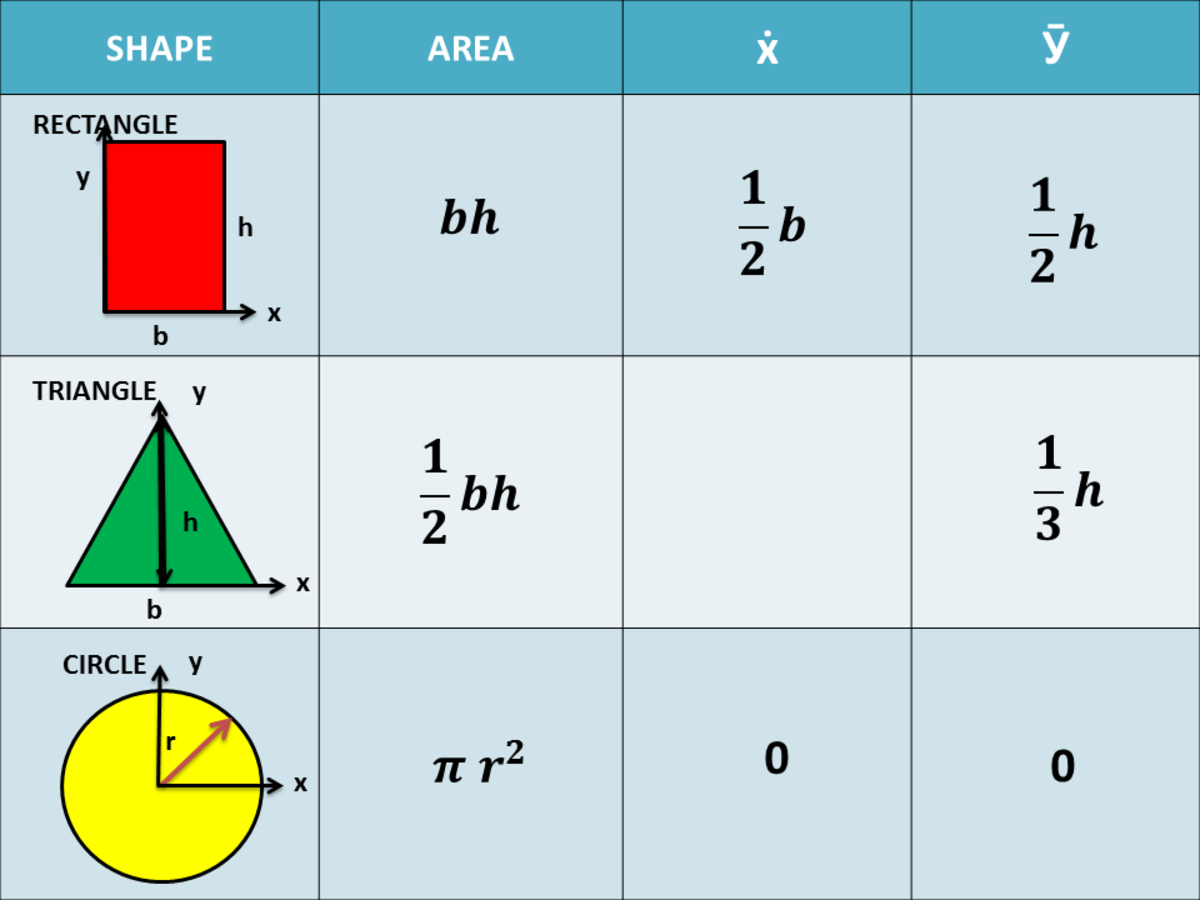Area and Centroid of Basic Shapes for the Computation of Moment of InertiaJohn Ray Cuevas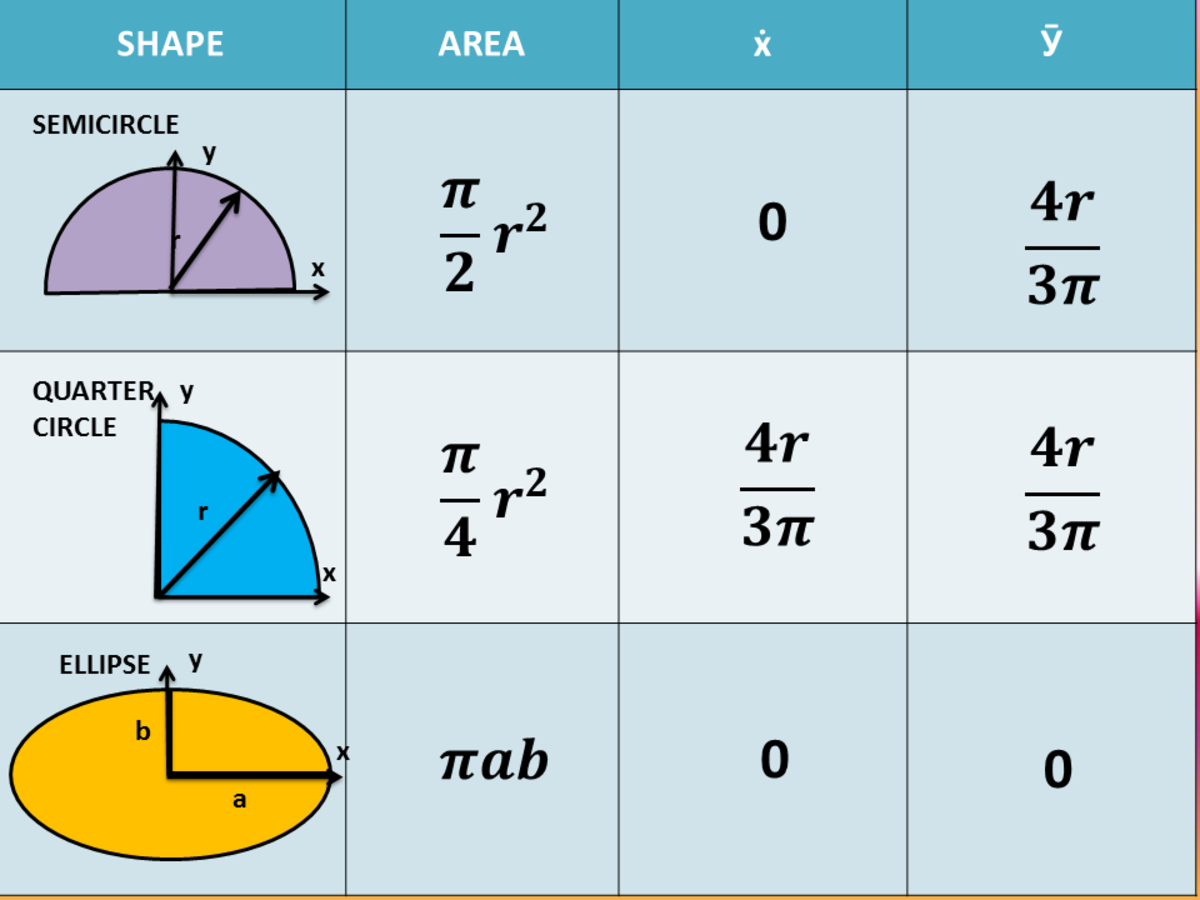Area and Centroid of Basic Shapes for the Computation of Moment of InertiaJohn Ray Cuevas

4. Once you obtained the location of the centroid from the axes, proceed to the calculation of the moment of inertia. Compute for the moment of inertia of each basic shape and refer for the formula for the basic shapes given below.

Below are the moment of inertia of basic shapes for its centroidal axis. To calculate the moment of inertia of a compound shape successfully, you must memorize the basic formula of the moment of inertia of basic geometric elements. These formulas are only applicable if the centroid of a basic shape coincides with the centroid of the irregular shape.

Scroll to Continue

5. If the centroid of the basic shape does not coincide, it is necessary to transfer the moment of inertia from that axis to the axis where the centroid of the compound shape is located using the 'Transfer Formula for Moment of Inertia'.

The moment of inertia with respect to any axis in the plane of the area is equal to the moment of inertia with respect to a parallel centroidal axis plus a transfer term composed of the product of the area of a basic shape multiplied by the square of the distance between the axes. The Transfer formula for Moment of Inertia is given below.

6. Get the summation of the moment of inertia of all basic shapes using transfer formula.

## Example 1: Square Hole Punch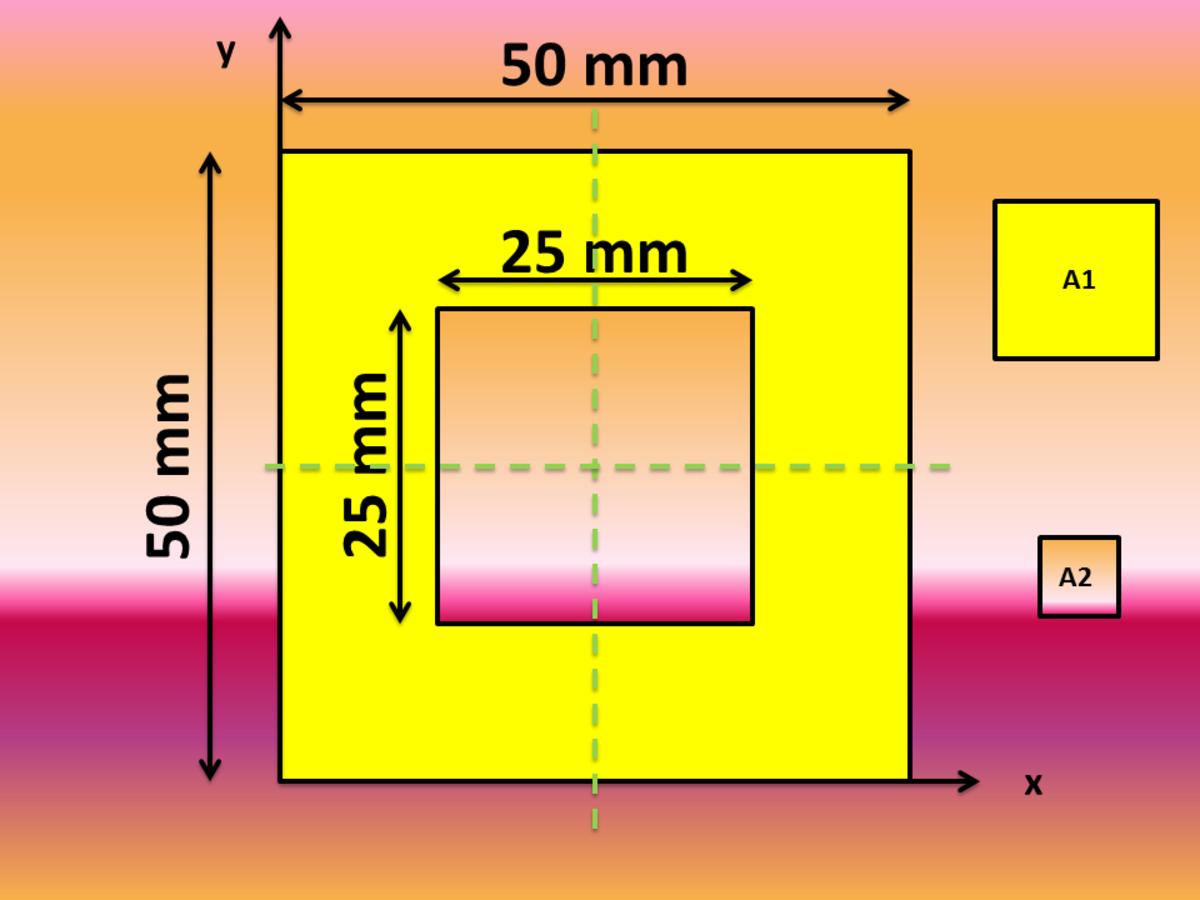Solving for the Moment of Inertia of Compound ShapesJohn Ray Cuevas

### Solution

a. Solve for the centroid of the whole compound shape. Since the figure is symmetrical in both directions, then its centroid is located on the middle of the complex figure.

b. Solve for the moment of inertia of the complex figure by subtracting the moment of inertia of area 2 (A2) from area 1 (A1). There is no need to use the transfer formula of moment of inertia since the centroid of all basic shapes coincide with the centroid of the compound shape.

## Example 2: C-Shape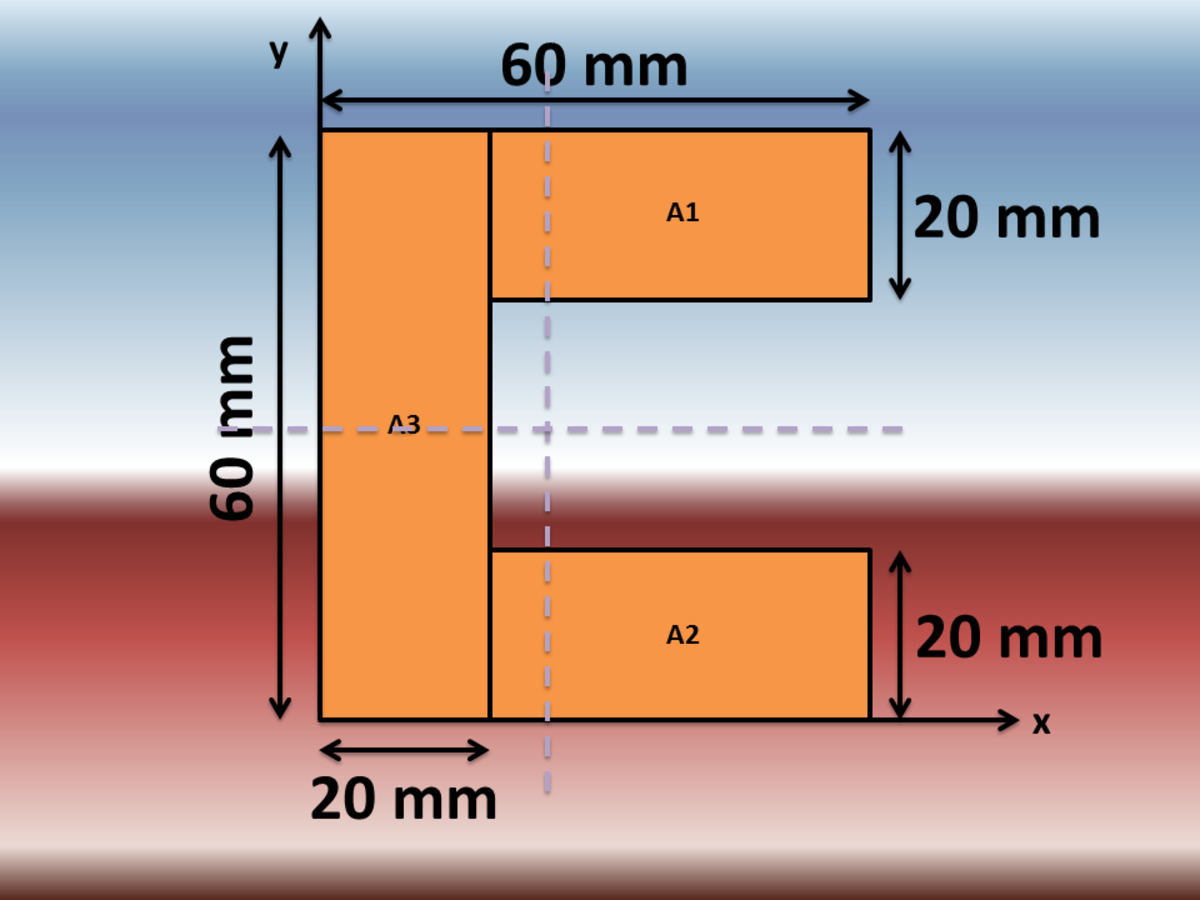Solving for the Moment of Inertia of Compound ShapesJohn Ray Cuevas

### Solution

a. Solve for the centroid of the whole complex shape by tabulating the solution.

LabelArea (mm^4)x-bar (mm)y-bar (mm)AxAy

A1

800

40

50

32000

40000

A2

800

40

10

32000

8000

A3

1200

10

30

12000

36000

TOTAL

2800

76000

84000

b. Solve for the moment of inertia using the transfer formula. The word "MOI" stands for Moment of Inertia.

## Example 3 - Snake Shape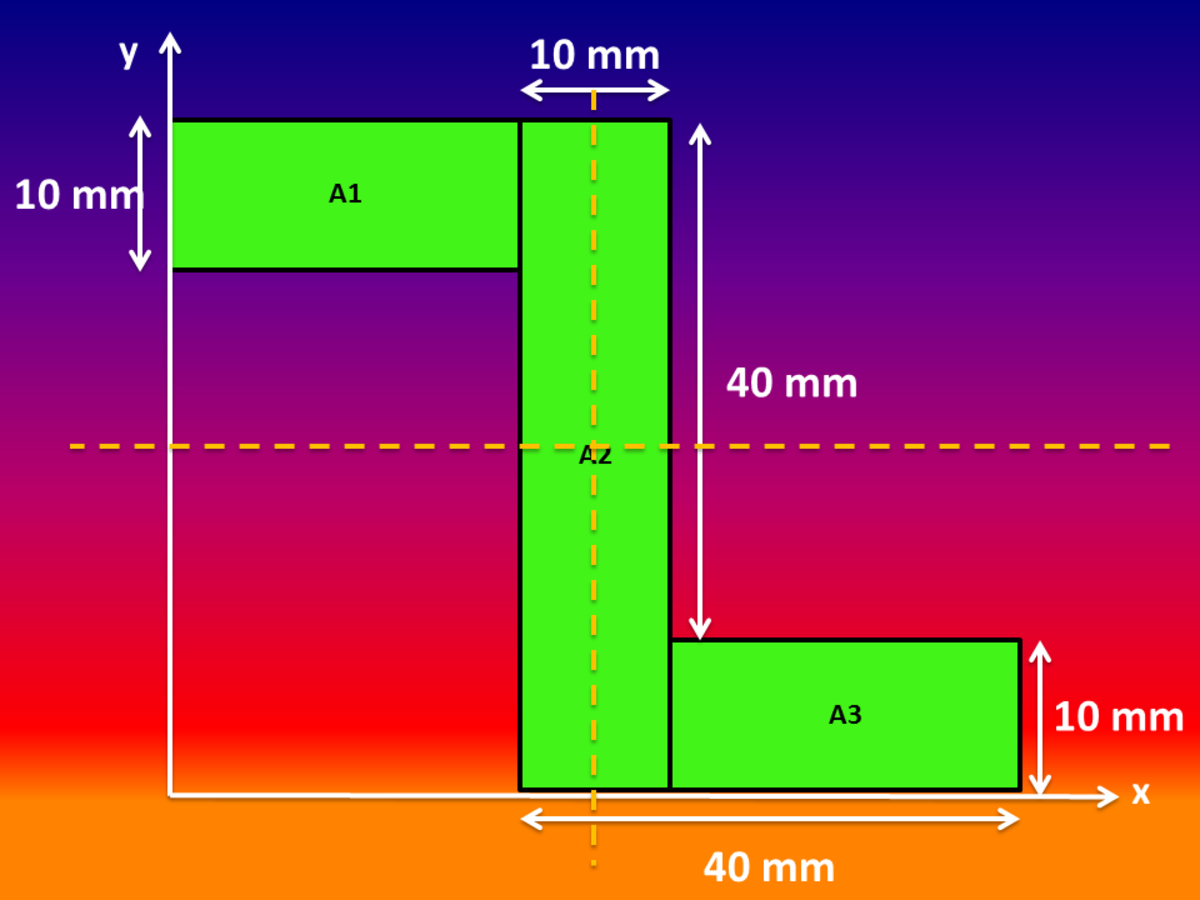Solving for the Moment of Inertia of Compound ShapesJohn Ray Cuevas

### Solution

a. Solve for the centroid of the whole complex shape by tabulating the solution.

LabelAreax-bar (mm)y-bar (mm)AxAy

A1

300

15

5

4500

1500

A2

500

35

25

17500

12500

A3

300

55

45

16500

13500

TOTAL

1100

38500

27500

b. Solve for the moment of inertia using the transfer formula. The word "MOI" stands for Moment of Inertia.

## Example 4: I-Shape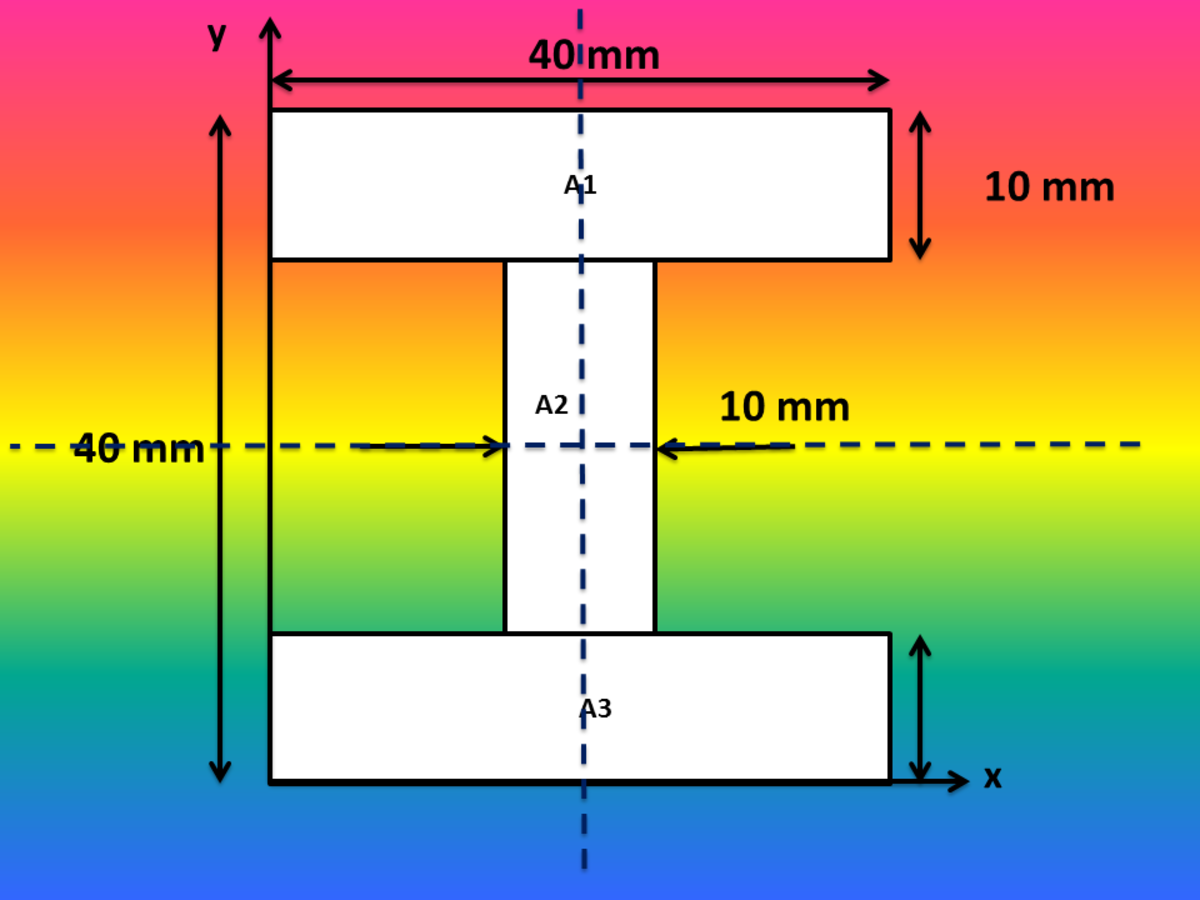Solving for the Moment of Inertia of Compound ShapesJohn Ray Cuevas

### Solution

a. Solve for the centroid of the whole compound shape. Since the figure is symmetrical in both directions, then its centroid is located on the middle of the complex figure.

b. Solve for the moment of inertia using the transfer formula. The word "MOI" stands for Moment of Inertia.

## Example 5: Complex Figure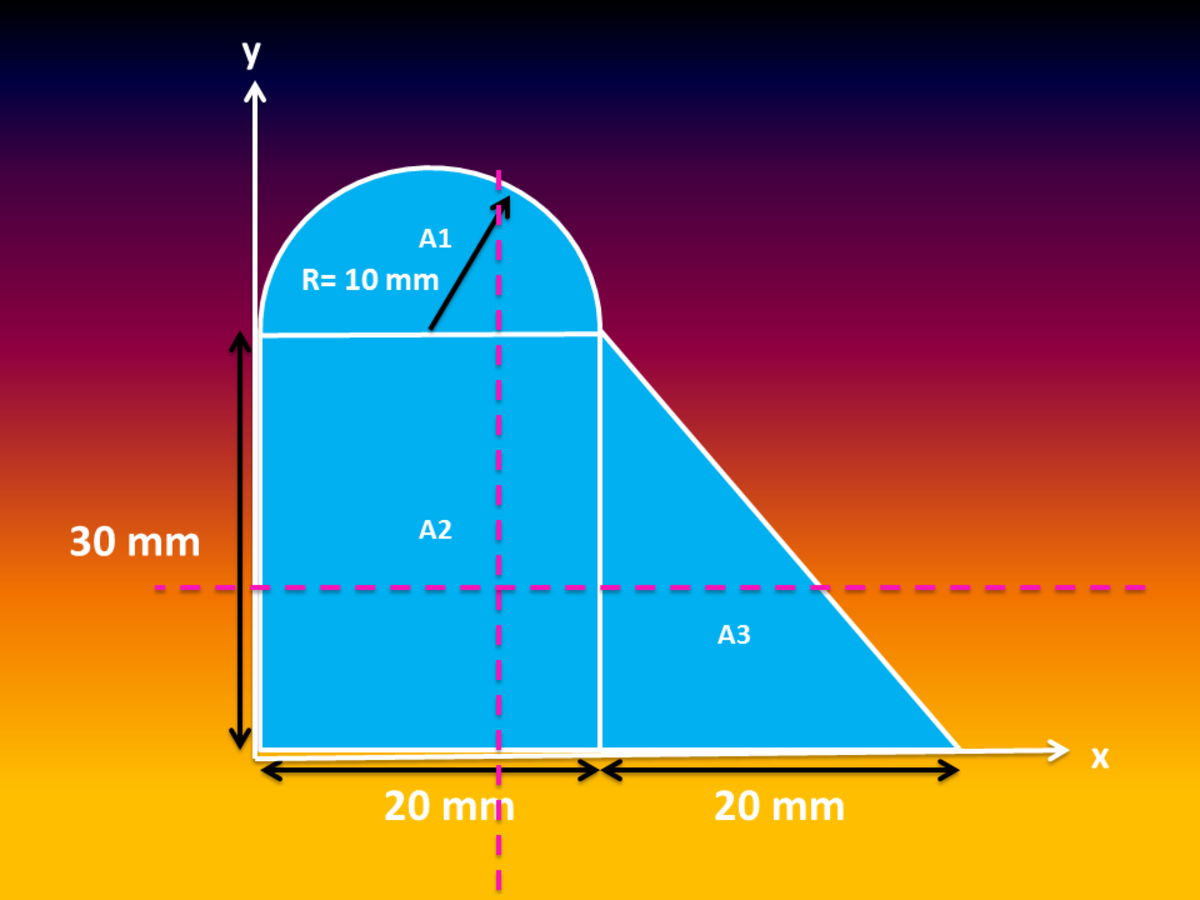Solving for the Moment of Inertia of Complex FiguresJohn Ray Cuevas

### Solution

a. Solve for the centroid of the whole complex shape by tabulating the solution.

LabelAreax-bar (mm)y-bar (mm)AxAy

A1

157.0796327

10

34.24413182

1570.796327

191.3237645

A2

600

10

15

6000

9000

A3

300

26.67

10

8001

3000

TOTAL

1057.079633

15571.79633

12191.32376

b. Solve for the moment of inertia using the transfer formula. The word "MOI" stands for Moment of Inertia.